Mathematics: CUET Mock Test - 5

# Mathematics: CUET Mock Test - 5

Test Description

## 40 Questions MCQ Test CUET Science Subjects Mock Tests | Mathematics: CUET Mock Test - 5

Mathematics: CUET Mock Test - 5 for Class 12 2023 is part of CUET Science Subjects Mock Tests preparation. The Mathematics: CUET Mock Test - 5 questions and answers have been prepared according to the Class 12 exam syllabus.The Mathematics: CUET Mock Test - 5 MCQs are made for Class 12 2023 Exam. Find important definitions, questions, notes, meanings, examples, exercises, MCQs and online tests for Mathematics: CUET Mock Test - 5 below.
Solutions of Mathematics: CUET Mock Test - 5 questions in English are available as part of our CUET Science Subjects Mock Tests for Class 12 & Mathematics: CUET Mock Test - 5 solutions in Hindi for CUET Science Subjects Mock Tests course. Download more important topics, notes, lectures and mock test series for Class 12 Exam by signing up for free. Attempt Mathematics: CUET Mock Test - 5 | 40 questions in 45 minutes | Mock test for Class 12 preparation | Free important questions MCQ to study CUET Science Subjects Mock Tests for Class 12 Exam | Download free PDF with solutions
 1 Crore+ students have signed up on EduRev. Have you?
Mathematics: CUET Mock Test - 5 - Question 1

### What is the name of the property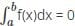?

Detailed Solution for Mathematics: CUET Mock Test - 5 - Question 1

The zero-length interval property is one of the properties used in definite integrals and they are always positive. The zero-length interval property is.

Mathematics: CUET Mock Test - 5 - Question 2

### Evaluate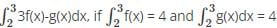.

Detailed Solution for Mathematics: CUET Mock Test - 5 - Question 2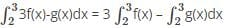= 3(4) – 4
= 8

Mathematics: CUET Mock Test - 5 - Question 3

### Compute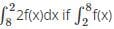= -3.

Detailed Solution for Mathematics: CUET Mock Test - 5 - Question 3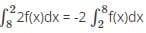= – 2(-3)
= 6

Mathematics: CUET Mock Test - 5 - Question 4

Evaluate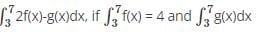= 2.

Detailed Solution for Mathematics: CUET Mock Test - 5 - Question 4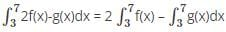= 2(4) – 2
= 6

Mathematics: CUET Mock Test - 5 - Question 5

Compute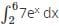.

Detailed Solution for Mathematics: CUET Mock Test - 5 - Question 5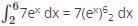= 7(e6 – e2)

Mathematics: CUET Mock Test - 5 - Question 6

Compute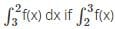= 4.

Detailed Solution for Mathematics: CUET Mock Test - 5 - Question 6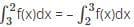= – 4

Mathematics: CUET Mock Test - 5 - Question 7

What property this does this equation come under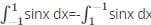?

Detailed Solution for Mathematics: CUET Mock Test - 5 - Question 7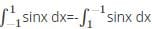comes under the reverse integral property.
In the reverse integral property the upper limits and lower limits are interchanged. The reverse integral property of definite integrals is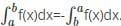Mathematics: CUET Mock Test - 5 - Question 8

What is the name of the property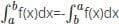?

Detailed Solution for Mathematics: CUET Mock Test - 5 - Question 8

In the reverse integral property the upper limits and lower limits are interchanged. The reverse integral property of definite integrals is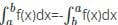.

Mathematics: CUET Mock Test - 5 - Question 9

Detailed Solution for Mathematics: CUET Mock Test - 5 - Question 9

The adding intervals property of definite integrals is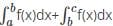.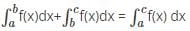Mathematics: CUET Mock Test - 5 - Question 10

What is the reverse integral property of definite integrals?

Detailed Solution for Mathematics: CUET Mock Test - 5 - Question 10

In the reverse integral property the upper limits and lower limits are interchanged. The reverse integral property of definite integrals is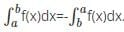Mathematics: CUET Mock Test - 5 - Question 11

If the order of the matrix is m×n, then how many elements will there be in the matrix?

Detailed Solution for Mathematics: CUET Mock Test - 5 - Question 11

The number of elements for a matrix with the order m × n is equal to mn, where m is the number of rows and n is the number of columns in the matrix.

Mathematics: CUET Mock Test - 5 - Question 12

What is the order of the matrix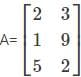?

Detailed Solution for Mathematics: CUET Mock Test - 5 - Question 12

The given matrixhas 3 rows and 2 columns. Therefore, the order of the matrix is 3×2.

Mathematics: CUET Mock Test - 5 - Question 13

Does Rolle’s theorem applicable if f(a) is not equal to f(b)?

Detailed Solution for Mathematics: CUET Mock Test - 5 - Question 13

According to Rolle’s theorem, if f : [a,b] → R is a function such that

• f is continuous on [a,b]
• f is differentiable on (a,b)
• f(a) = f(b) then there exists at least one point c ∈ (a,b) such that f’(c) = 0
Mathematics: CUET Mock Test - 5 - Question 14

Another form of Rolle’s theorem for the continuous condition is _____

Detailed Solution for Mathematics: CUET Mock Test - 5 - Question 14

According to Rolle’s theorem, if f : [a,a+h] → R is a function such that

• f is continuous on [a,a+h]
• f is differentiable on (a,a+h)
• f(a) = f(a+h) then there exists at least one θ c ∈ (0,1) such that f’(a+θh) = 0
Mathematics: CUET Mock Test - 5 - Question 15

The matrix which follows the conditions m=n is called?

Detailed Solution for Mathematics: CUET Mock Test - 5 - Question 15

A square matrix is a matrix in which the number of rows(m) is equal to the number of columns(n). Therefore, the matrix which follows the condition m=n is a square matrix.

Mathematics: CUET Mock Test - 5 - Question 16

The matrix which follows the condition m>n is called as ____________

Detailed Solution for Mathematics: CUET Mock Test - 5 - Question 16

The matrix in which the number of columns is greater than the number of rows is called a vertical matrix. There the matrix which follows the condition m>n is a vertical matrix.

Mathematics: CUET Mock Test - 5 - Question 17

Rolle’s theorem is a special case of _____

Detailed Solution for Mathematics: CUET Mock Test - 5 - Question 17

Rolle’s theorem is just a special case of Lagrange’s mean value theorem when f(a) = f(b) and Lagrange’s mean value theorem is also called the mean value theorem.

Mathematics: CUET Mock Test - 5 - Question 18

What is the formula for Lagrange’s theorem?

Detailed Solution for Mathematics: CUET Mock Test - 5 - Question 18

According to Lagrange’s mean value theorem, if f : [a,b] → R is a function such that f is differentiable on (a,b) then the formula for Lagrange’s theorem is f’(c) =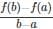.

Mathematics: CUET Mock Test - 5 - Question 19

Function f should be _____ on [a,b] according to Rolle’s theorem.

Detailed Solution for Mathematics: CUET Mock Test - 5 - Question 19

According to Rolle’s theorem, if f : [a,b] → R is a function such that

• f is continuous on [a,b]
• f is differentiable on (a,b)
• f(a) = f(b) then there exists at least one point c ∈ (a,b) such that f’(c) = 0
Mathematics: CUET Mock Test - 5 - Question 20

What is the relation between f(a) and f(b) according to Rolle’s theorem?

Detailed Solution for Mathematics: CUET Mock Test - 5 - Question 20

According to Rolle’s theorem, if f : [a,b] → R is a function such that

• f is continuous on [a,b]
• f is differentiable on (a,b)
• f(a) = f(b) then there exists at least one point c ∈ (a,b) such that f’(c) = 0
Mathematics: CUET Mock Test - 5 - Question 21

Another form of Rolle’s theorem for the differential condition is _____

Detailed Solution for Mathematics: CUET Mock Test - 5 - Question 21

According to Rolle’s theorem, if f : [a,a+h] → R is a function such that

• f is continuous on [a,a + h]
• f is differentiable on (a,a + h)
• f(a) = f(a + h) then there exists at least one θ c ∈ (0,1) such that f’(a + θh) = 0
Mathematics: CUET Mock Test - 5 - Question 22

What is the relation between f(a) and f(h) according to another form of Rolle’s theorem?

Detailed Solution for Mathematics: CUET Mock Test - 5 - Question 22

According to Rolle’s theorem, if f : [a, a + h] → R is a function such that

• f is continuous on [a, a + h]
• f is differentiable on (a,a+h)
• f(a) = f(a + h) then there exists at least one θ c ∈ (0,1) such that f’(a + θh) = 0
Mathematics: CUET Mock Test - 5 - Question 23

What are/is the conditions to satify Lagrange’s mean value theorem?

Detailed Solution for Mathematics: CUET Mock Test - 5 - Question 23

According to Lagrange’s mean value theorem, if f : [a,b] → R is a function such that

• f is continuous on [a,b]
• f is differentiable on (a,b) then there exists a least point c ∈ (a,b) such that f’(c) =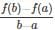.
Mathematics: CUET Mock Test - 5 - Question 24

Lagrange’s mean value theorem is also called as _____

Detailed Solution for Mathematics: CUET Mock Test - 5 - Question 24

Lagrange’s mean value theorem is also called the mean value theorem and Rolle’s theorem is just a special case of Lagrange’s mean value theorem when f(a) = f(b).

Mathematics: CUET Mock Test - 5 - Question 25

Is Rolle’s theorem applicable to f(x) = tan x on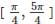?

Detailed Solution for Mathematics: CUET Mock Test - 5 - Question 25

Given function is f(x) = tan x onF(x) = tan x is not defined at x onSo, f(x) is not continuous on.
Hence, Rolle’s theorem is not applicable.

Mathematics: CUET Mock Test - 5 - Question 26

Find ’C’ using Lagrange’s mean value theorem, if f(x) = ex, a = 0, b = 1.

Detailed Solution for Mathematics: CUET Mock Test - 5 - Question 26

Given f(x) = ex, a = 0, b = 1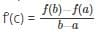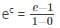ec = e - 1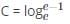Mathematics: CUET Mock Test - 5 - Question 27

Find the derivative of f(x) = sin(x2).

Detailed Solution for Mathematics: CUET Mock Test - 5 - Question 27

Differentiation of the function f(x) = sin(x2) is done with chain rule. First we differentiate sin function which becomes cos and then differentiate the inner (x2) which becomes 2x, hence it comes out to be 2xcos(x2).

Mathematics: CUET Mock Test - 5 - Question 28

Find derivative of tan(x+4).

Detailed Solution for Mathematics: CUET Mock Test - 5 - Question 28

We know that derivative of tanx is sec2(x), now in the above question we get tan(x + 4), hence its derivative comes out to be sec2(x + 4), as the inside expression (x + 4) is differentiated into 1.
Therefore the answer is sec2(x + 4).

Mathematics: CUET Mock Test - 5 - Question 29

Value after differentiating cos (sinx) is _________

Detailed Solution for Mathematics: CUET Mock Test - 5 - Question 29

We differentiate the given function with the help of chain rule so we first differentiate the outer function which becomes –sin and then we differentiate the inner function sinx which is differentiated and comes out to be cosx, hence the differentiated function comes out to be -sin (sinx).cosx.

Mathematics: CUET Mock Test - 5 - Question 30

Find dy/dx of 2x+3y = sinx.

Detailed Solution for Mathematics: CUET Mock Test - 5 - Question 30

Differentiating on both sides we get 2 + 3dy/dx = cosx.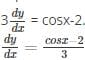Mathematics: CUET Mock Test - 5 - Question 31

If y =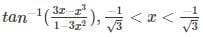Detailed Solution for Mathematics: CUET Mock Test - 5 - Question 31

Given function is y =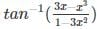Now taking RHS and substituting x = tang in it and then we get,
y =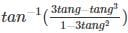, Now it becomes the expansion of the function tan3g,
Hence the given function becomes y= tan-1(tan3g). Which is equal to 3g, now substituting the value of g= tan-1x, now after differentiating both sides we get the answer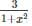Mathematics: CUET Mock Test - 5 - Question 32

What is derivative of xn?

Detailed Solution for Mathematics: CUET Mock Test - 5 - Question 32

It is a standard rule for derivative of a function of this form in which the original power comes in front and the value in the power is decreased by one. Therefore the only option of this form is nxn-1 from the given options.

Mathematics: CUET Mock Test - 5 - Question 33

What is value of dy/dx if x - y = 1?

Detailed Solution for Mathematics: CUET Mock Test - 5 - Question 33

We know x-y = 1, hence we differentiate it on both sides:
We get 1- dy/dx = 0, dy/dx = 1, hence the value of dy/dx comes out to be 1.

Mathematics: CUET Mock Test - 5 - Question 34

Value after differentiating cos (x+ 5) is ________

Detailed Solution for Mathematics: CUET Mock Test - 5 - Question 34

We differentiate the given function with help of chain rule and hence the outer function becomes –sin and the inner function is differentiated into 2x, therefore the answer comes out to be -sin (x2 + 5).2x.

Mathematics: CUET Mock Test - 5 - Question 35

What is derivative of cotx?

Detailed Solution for Mathematics: CUET Mock Test - 5 - Question 35

The derivative of cotx is –cosec2x, as this function has a fixed derivative like sinx has its derivative cosx. Therefore the answer to the above question is –cosec2x.

Mathematics: CUET Mock Test - 5 - Question 36

Find dy/dx of y = sin (ax + b).

Detailed Solution for Mathematics: CUET Mock Test - 5 - Question 36

We differentiate the given function with the help of chain rule and hence
the outer function is differentiated into cos, and the inner function comes out to be a and the constant b becomes 0, which is multiplied to the whole function and the answer comes out to be
⇒ a.cos (ax + b).

Mathematics: CUET Mock Test - 5 - Question 37

What is the mathematical expression for the definition of continuity?

Detailed Solution for Mathematics: CUET Mock Test - 5 - Question 37

function f defined on (a,b) is said to be continuous on (a,b) if it is continuous at every point of (a,b) i.e., if limx→c⁡f(x) = f(c) ∀ c ∈ (a,b).

Mathematics: CUET Mock Test - 5 - Question 38

What is the mathematical expression for f is right continuous on (a,b)?

Detailed Solution for Mathematics: CUET Mock Test - 5 - Question 38

A function is said to be continuous when it is both left continuous and right continuous. Mathematical expression for a function f is right continuous on (a,b) is limx→a+⁡f(x)=f(a).

Mathematics: CUET Mock Test - 5 - Question 39

Which of the following is not a type of matrix?

Detailed Solution for Mathematics: CUET Mock Test - 5 - Question 39

Minor matrix is not a type of matrix. Scalar, diagonal, symmetric are various type of matrices.

Mathematics: CUET Mock Test - 5 - Question 40

What is/are conditions for a function to be continuous on (a,b)?

Detailed Solution for Mathematics: CUET Mock Test - 5 - Question 40

The three conditions required for a function f is said to be continuous on (a,b) if f is continuous at each point of (a,b), f is right continuous at x = a, f is left continuous at x = b.

## CUET Science Subjects Mock Tests

60 tests
 Use Code STAYHOME200 and get INR 200 additional OFF Use Coupon Code
Information about Mathematics: CUET Mock Test - 5 Page
In this test you can find the Exam questions for Mathematics: CUET Mock Test - 5 solved & explained in the simplest way possible. Besides giving Questions and answers for Mathematics: CUET Mock Test - 5, EduRev gives you an ample number of Online tests for practice

60 tests